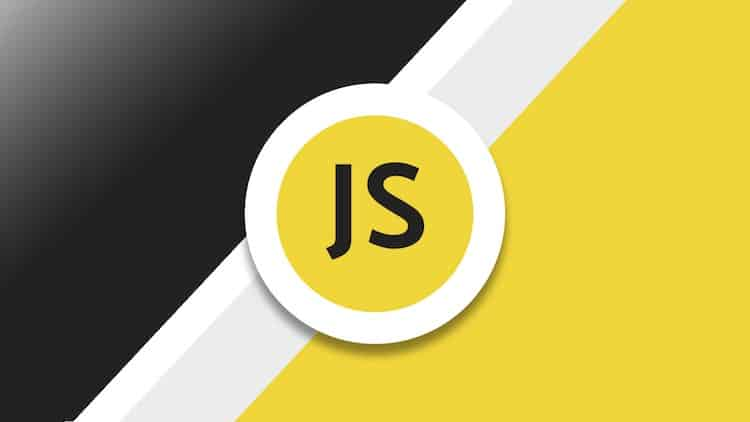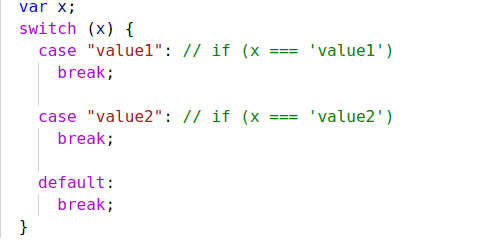December 27, 2019 - 12:36 pm

# The Fundamentals of JavaScript (Final Part)

We have discussed the fundamentals of JavaScript in my previous two blogs. Today I am going to discuss the rest of the fundamentals of JavaScript, and this is the final part of fundamentals series. Today we cover Switch case, Function, Arrow function, and JavaScript Special. Let’s get started.

#### Switch Statement :

A Switch case statement can replace multiple if checks in a more descriptive way.

#### The Syntax :

The switch case has one or more cases with a default option. Here is the syntax:The value of x is checked for strict equality to the value from the first case that is value 1 after that check value 2 and so on. If there the equality found then switch case start to execute code and after executing code unit execute break. If no equality found, then, it executes the default case.

#### Grouping of Case :

If we want to execute multiple cases for the same value, we will use the Grouping case.

Take a look at the following example:

Now Case 3 and 5 show the same message. The ability to group we can know-how switch casework without break statement.

### Function

Sometimes we need to use the same piece of code multiple times in the script. For example, in a script, we need to add two numbers multiple times. For that, we will write a function that takes two numbers as a parameter return sum of two numbers.

#### Function Declaration:

When we call the function like showMessage any part of the same function, showMessage function execute and show the alert.

#### Local and Global variables :

Variable that declare inside any function that called a local variable, because we can not access those variables outside of this function.

A global variable that declared the outer side of the function, we can use those variables inside a function and all over in the script.

There we can get clear the concept of global and local variables. In the first function message a local variable (Declare inside a function) inside a function, console log the message, But outside the function, it will through an error because we can not access a local variable outside of the function. But we can access the global variable (Function 2 UserName) inside and outside any function and modify the value inside function.

#### Parameters:

We can pass arbitrary data to function using parameters.

When the function is called in lines (**) the given values are copied to local variables from and text. Then the function uses them.

#### Returning Value:

A function can return value to the code wherefrom it called

Add Numbers function returns the sum of two number 1, and 3. We can save the value in a variable x. This way a function can return value.